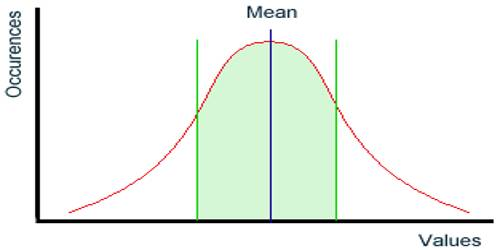Statistical Mean

The statistical mean refers to the mean or average that is used to derive the central tendency of the data in question. It is determined by adding all the data points in a population and then dividing the total by the number of points. The resulting number is known as the mean or the average.

For example, the mean mark obtained by students in a test is required to correctly gauge the performance of a student in that test. If the student scores a low percentage but is well ahead of the mean, then it means the test is difficult and therefore his performance is good, something that simply a percentage will not be able to tell.

In statistics, the mean is the average of a set of data. To find the mean, sum all the numbers and then divide by the number of items in the set. For example, to find the mean of the following set of numbers: 21, 23, 24, 26, 28, 29, 30, 31, 33

21 + 23 + 24 + 26 + 28 + 29 + 30 + 31 + 33 = 245

Then divide your answer by the number of items in your set. There are 9 numbers, so:

245 / 9 = 27.222

The statistical mean is commonly called the average.

Another Example:

For example, let’s say you have four test scores: 15, 18, 22, and 20. To find the average, you would first add all four scores together, then divide the sum by four. The resulting mean is 18.75. Written out, it looks something like this:

(15 + 18 + 22 + 20) / 4 = 75 / 4 = 18.75

If you were to round up to the nearest whole number, the average would be 19.To find the mean of a group of numbers: